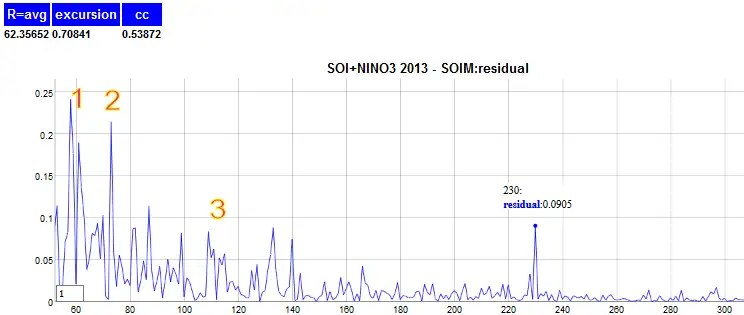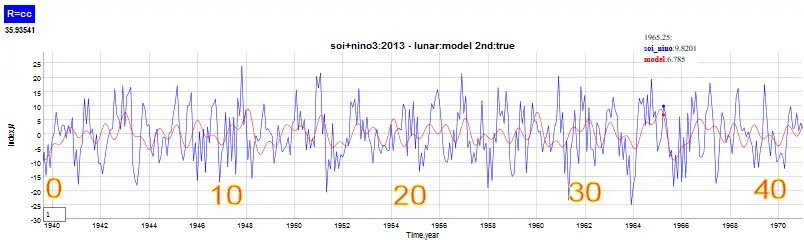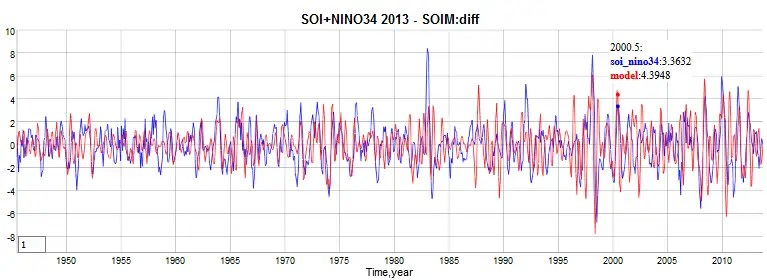# Tidal Locking and ENSO

As I have been formulating a model for ENSO, I always try to relate it to a purely physical basis. The premise I have had from the beginning is that some external factor is driving the forcing of the equatorial Pacific thermocline. This forcing stimulus essentially causes a sloshing in the ocean volume due to small changes in the angular momentum of the rotating earth. I keep thinking that the origin is lunar as the success of the QBO model in relating lunisolar forcing to the oscillatory behavior of the QBO winds is enough motivation to keep on a lunar path.

Yet, I am finding that the detailed mechanism for ENSO differs from that of QBO. An interesting correlation I found is in the tidal-locking of the Earth to the moon. I think this is a subset of the more general case of spin-orbit resonance, where the rotation rate of a satellite is an integral ratio of the main body. In the case of the moon and the earth, it explains why the same moon face is always directed at the earth — as they spin at the same rate during their mutual orbit, thus compensating via a kind of counter-rotation as shown in the left figure below.Fig 1: Tidal Locking (left) results in the spin of the moon

The reason that they spin at the same rate is like most physical behaviors explainable via classical mechanics and the principle of least action. In essence, if one body slows down, the other will slow in response.

If the earth and moon spin at a uniform rate, there is no means for changes in angular momentum to get imparted to the Pacific ocean. And so a spinning planet is not enough to induce a physical sloshing. This is probably too obvious an assertion, but if you just hold a glass of water, it won’t start sloshing just because the earth is rotating underneath it. So we can make an assumption that the initial momentum changes needed for the earth to get up to speed have long since equilibrated.

The question is whether there is an obvious modulation of the moon’s rotation rate that could impart subtle angular momentum shifts in the earth’s own spin. This would quickly get absorbed in the sensitive response of ocean sloshing. As described in ref  below, the solid moon does show a longitudinal modulation (i.e. libration) at a period of 1095 days (due to the earth-moon-sun interaction of 1/2 of the perigee cycle ~3 years) and a free resonant period of 1056 days, or ~2.89 years.

Does that latter period show up in the ENSO forcing model? Yes, and it is a very strong and sharp forcing factor in the wave-equation ENSO model, also see this comment. But what is even more substantiating for the idea is that an induced seasonal harmonic is also seen in the power spectra of the ENSO time series. This value should be at 1/(1+1/2.89) = 0.743 years if the yearly cycle modulates the lunar libration. The other cycle should be at 1/(1-1/2.89) = 1.53 years. In the following figure, which is a power spectra of the residual from the ENSO model, a clear sharp peak can be seen in the 2048 point monthly Fourier Transform at wave number 230. Seen in the Figure 2 below, sitting all by its lonesome but rising distinctly from the higher frequency ENSO noise is a peak corresponding to a period of 2048/12/230=0.742 years!Fig 2: Seasonal modulation with the moon’s free libration rate aligns with wavenumber 230

In the figure, the other labeled points each have a meaning. Peak 1 is the residual of the 2.89 year factor, split into two and reduced in magnitude after being included in the model. Peak #2 is likewise the ubiquitous 2.33 year period model reduced. And the peaks at #3 are centered nearly at a period corresponding to 1.53 years, which is the factored complement to the 0.743 year libration modulation period.

The other relation is that 2.89 years is very close to the Chandler wobble of ~6.5 years modulated by the biennial cycle, so that this relation holds.
1/2.89 + 1/6.5 = 1/2

Which means that a period of 1/(1/2+1/6.5) = 1.53 would show up around peak #3. Its possible that the lunar libration of 2.9 years is working with the chandler wobble to induce a metastable biennial locking to ENSO.

Figure 3 is a segment of a multiple regression fit to the second derivative showing how the libration modulation emerges. The modulation is subtle but significant as a count of 40 cycles of 0.742 year period spans that 30 year interval.Fig 3: The 0.742 year period extracted via multiple linear regression

Figure 4 is a similar plot but the 2nd derivative was applied after a wave-equation DiffEq solution was fitted to the ENSO time series. Since the 1940’s the amount of noise in the ENSO measures has been reduced so that correlation is obvious.Fig 4: The 2nd derivative of the ENSO signal aligned with the wave-equation model solution fit.

With the biennial mode in place, it appears that most of the significant ENSO peaks appear as pairs separated by the +/- sideband split from either a yearly or biennial modulation applied.

Early on I put some effort into analyzing the Mathieu equation formulation of the wave equation, and how it is applied to describe sloshing. So it’s interesting to note how a parameterization of the Mathieu equation can mimic the behavior of a modulated forcing.Fig. 5 : (Left) Mathieu equation featuring a biennial modulation on the LHS
(Right) Wave equation featuring a biennial modulation of the RHS forcing. The responses are very similar.

So it is still possible that the biennial forcing we are seeing could potential be a property of the ocean volume, thus providing an alternative interpretation via the full Mathieu wave equation. The key remains to be able to fully characterize and understand the phase reversal of the biennial mode between the years 1980 and 1996. The quality of the modeling fits crucially depend on this being a real phenomenon.

## References

 Rambaux, N., and J. G. Williams. “The Moon’s physical librations and determination of their free modes.” Celestial Mechanics and Dynamical Astronomy 109.1 (2011): 85-100.

## 2 thoughts on “Tidal Locking and ENSO”

1.admin

This team is asserting similar claims for ENSO forcing

They claim the distribution of periodic factors leads to a non-chaotic behavior

Like